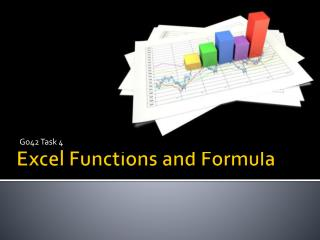DownloadDownload PresentationExcel Functions and Formula

# Excel Functions and Formula

Download Presentation## Excel Functions and Formula

- - - - - - - - - - - - - - - - - - - - - - - - - - - E N D - - - - - - - - - - - - - - - - - - - - - - - - - - -
##### Presentation Transcript

1. G042 Task 4 Excel Functions and Formula

2. Session Objectives • Understand how to use a range of different functions in excel. • Used a range of functions within your excel spreadsheet.

3. Sum Function • The syntax for sum function =Sum(first cell:last cell)

4. Max or Min Function • The syntax for max function =Max(first cell:last cell) • The syntax for max function =Min(first cell:last cell)

5. Average (Mean) Function • The syntax for average function =Average (first value, second value, etc.)

6. Other Averages • To work out the median =Median (first value, second value, etc.) • To work out the mode =Mode (first value, second value, etc.)

7. Count Function • The syntax for sum function =Count(first cell:last cell) • Blank entries are not counted. • Text entries are NOT counted.

8. CountA Function • The syntax for sum function =CountA(first cell:last cell) • Blank entries are not counted. • Text entries are counted.

9. IF Function • The syntax is =IF (condition, value-if-true, value-if-false) • value returned may be either a number or text • if value returned is text, it must be in quotes

10. CountIf Function • The syntax for sum function =CountIf(first cell:last cell, criteria)

11. Int Function • Int returns the nearest integer • The syntax for Int function =Int(number)

12. LOOKUP Functions • Returns a value from a list • The syntax for VLOOKUP function =VLOOKUP(lookup_value, table_array, column where return data is, false)

13. Function Wizard • In excel there is a help tool for functions called the function wizard. • There are two ways to get the the function wizard. If you look at the standard toolbar, the function wizard icon look like the icon on the right. • The other way to get to the function wizard is to go to the menu INSERT -- down to FUNCTION.

14. Next Steps • Add a range of useful functions to your spreadsheet – think why you are going to include them – don’t just include for the sake of it.

15. Help Sources • Online tutorials • Help In Excel (PRESS f1)